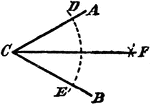### Construction Of A Divided Angle

An illustration showing the construction used to divide an angle into two equal parts. "With C as a…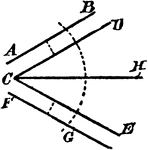### Construction Of A Divided Angle

An illustration showing the construction used to divide an angle into two equal parts when the lines…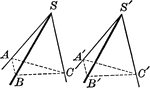### Equal Polyhedral Angles

Illustration of two polyhedral angles that are equal.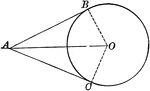### Circle With Two Tangents Drawn From an External Point

Illustration of a circle which illustrates that the tangents to a circle drawn from an external point…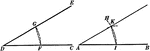### Construction Of An Equal Angle

Illustration used to show how to construct an angle equal to a given angle when given a vertex and a…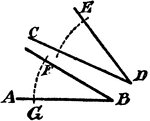### Construction Of An Equal Angle

An illustration showing the construction used to erect an equal angle. "With D as a center, draw the…